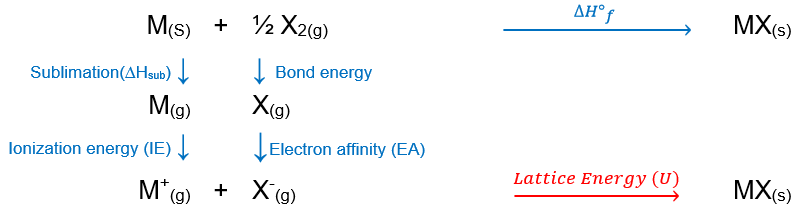# Problem: Consider an ionic compound, MX, composed of generic metal M and generic, gaseous halogen X.The enthalpy of formation of MX is ΔHf° = -453 kJ/mol. The enthalpy of sublimation of M is ΔHsub = 127 kJ/mol. The ionization energy of M is IE = 431 kJ/mol. The electron affinity of X is ΔHEA = -301 kJ/mol. The bond energy of X2 is BE = 171 kJ/mol.Determine the lattice energy of MX____ kJ/mol

###### FREE Expert Solution

Recall that lattice energy is the energy required to combine two gaseous ions into a solid ionic compound:

M+(g) + X(g)  MX(s)

To calculate for lattice energy, we need to do the Born-Haber cycle for MX

We start with the corresponding formation equation for MX:

M(s) + ½ X2(g)  MX(s), ΔH˚f92% (4 ratings)###### Problem Details

Consider an ionic compound, MX, composed of generic metal M and generic, gaseous halogen X.

The enthalpy of formation of MX is ΔHf° = -453 kJ/mol. The enthalpy of sublimation of M is ΔHsub = 127 kJ/mol. The ionization energy of M is IE = 431 kJ/mol. The electron affinity of X is ΔHEA = -301 kJ/mol. The bond energy of X2 is BE = 171 kJ/mol.

Determine the lattice energy of MX

____ kJ/mol

Frequently Asked Questions

What scientific concept do you need to know in order to solve this problem?

Our tutors have indicated that to solve this problem you will need to apply the Born Haber Cycle concept. You can view video lessons to learn Born Haber Cycle. Or if you need more Born Haber Cycle practice, you can also practice Born Haber Cycle practice problems.